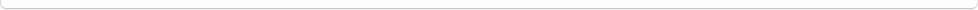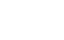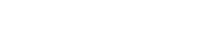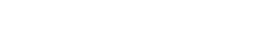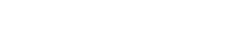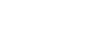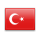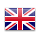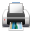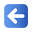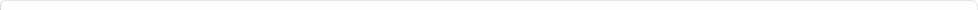Description of Individual Course Units
 Course Unit Code Course Unit Title Type of Course Unit Year of Study Semester Number of ECTS Credits 140400106104 MATHEMATICAL ECONOMICS Elective 3 6 4
Level of Course Unit
First Cycle
Objectives of the Course
A course on "Mathematical Economics" aims to give students necessary analytical tools and skills of Mathematics that help to understand and solve economic models like market model, national income model, etc.
Name of Lecturer(s)
Learning Outcomes
 1 To be able to determine the endogenous and exogenous variables of economic models. 2 To be able to determine the endogenous and exogenous variables of economic models. 3 To be able to solve the equation systems for the equilibrium analysis. 4 To be able to solve the equation systems for the equilibrium analysis. 5 To be able to use matrix algebra in order to solve the linear equation systems. 6 To be able to use matrix algebra in order to solve the linear equation systems. 7 Learn the use of differentiation techniques for the comparative static analysis. 8 Learn the use of differentiation techniques for the comparative static analysis. 9 To be able to determine the optimum values of an economic variable in non-constraint and constraint cases. 10 To be able to determine the optimum values of an economic variable in non-constraint and constraint cases.
Mode of Delivery
Daytime Class
Prerequisites and co-requisities
NONE
Recommended Optional Programme Components
Course Contents
Equilibrium analysis, linear models and matrix algebra, rules of differentiation and their use in comparative statics, optimization problems.
Weekly Detailed Course Contents
 Week Theoretical Practice Laboratory 1 Chapter 1 & Chapter 2: The Nature of Mathematical Economics & Economic Models 2 Chapter 3: Equilibrium Analysis in Economics 3 Chapter 3: Equilibrium Analysis in Economics 4 Chapter 4: Linear Models and Matrix Algebra 5 Chapter 4: Linear Models and Matrix Algebra 6 Chapter 5: Linear Models and Matrix Algebra (continued) 7 Chapter 5: Linear Models and Matrix Algebra (continued) 8 9 Chapter 7: Rules of Differentiation and Their Use in Comparative Statistics 10 Chapter 7: Rules of Differentiation and Their Use in Comparative Statistics 11 Chapter 9: Optimization: A Special Variety of Equilibrium Analysis 12 Chapter 9: Optimization: A Special Variety of Equilibrium Analysis 13 Chapter 12: Optimization with Equality Constraints 14 Chapter 12: Optimization with Equality Constraints